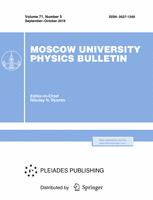Faculty of Physics
M.V.Lomonosov Moscow State University
Regular Article

# Wind waves evolution in the annular channel

## N.P. Savenkova$^1$, S.A. Skladchikov$^1$, V.S. Laponin$^1$, S.V. Anpilov$^1$, V. Yusupaliev$^2$, S.A. Shuteev$^2$

### Memoirs of the Faculty of Physics 2015. N 4.

• Article
Annotation

Currently not enough investigated both experimentally and theoretically the ratio between the length, amplitude and velocity of such waves. For this reason, this work is devoted to the study of these relations by means of mathematical modeling.

PACS:
47.35.Lf Wave-structure interactions
Authors
N.P. Savenkova$^1$, S.A. Skladchikov$^1$, V.S. Laponin$^1$, S.V. Anpilov$^1$, V. Yusupaliev$^2$, S.A. Shuteev$^2$
$^1$ Department of mathematical modeling in physics, Faculty of Computational mathematics and cybernetics, Lomonosov Moscow State University. Moscow 119991, Russia.
$^2$ Department of Physical Research, Faculty of Physics, Lomonosov Moscow State University. Moscow 119991, Russia.### Moscow University Physics Bulletin### Science News of the Faculty of Physics, Lomonosov Moscow State University

This new information publication, which is intended to convey to the staff, students and graduate students, faculty colleagues and partners of the main achievements of scientists and scientific information on the events in the life of university physicists.